# Quasi-regular mapping

mapping with bounded distortion

Letbe an open connected subset of the Euclidean space,, and let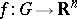be a continuous mapping of Sobolev class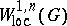(cf. also Sobolev space). Thenis said to be quasi-regular if there is a number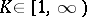such that the inequalityis satisfied almost everywhere (a.e.) inHere,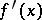denotes the formal derivative ofat, i.e. the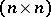-matrix of partial derivatives of the coordinate functions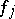of. Further,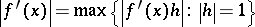and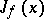is the Jacobian determinant (cf. also Jacobian) ofat. The smallest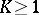in the above inequality is called the outer dilatation ofand is denoted by. Ifis quasi-regular, then the inner dilatation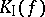is the smallest constantin the inequality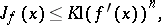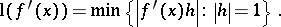The maximal dilatation is the number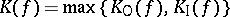, and one says thatis-quasi-regular if.

## Particular cases.

For the dimensionand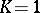, the class of-quasi-regular mappings agrees with that of the complex-analytic functions (cf. also Analytic function). Injective quasi-regular mappings in dimensionsare called quasi-conformal (see Quasi-conformal mapping; [a4], [a8], [a10]). Forand, a-quasi-regular mapping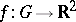can be represented in the form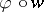, where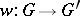is a-quasi-conformal homeomorphism and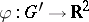is an analytic function (Stoilow's theorem). There is no such representation in dimensions. Note that for all dimensionsand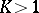the set of those points where a-quasi-conformal mapping is not differentiable may be non-empty and the behaviour of the mapping may be very curious at the points of this set, which has also Lebesgue measure zero. Thus, there is a substantial difference between the two casesand.

For higher dimensionsthe theory of quasi-regular mappings is essentially different from the plane case. There are several reasons for this:

a) there are neither general existence theorems nor counterparts of power series expansions in higher dimensions;

b) the usual methods of function theory are not applicable in the higher-dimensional setup;

c) in the plane case the class of conformal mappings is very rich (cf. also Conformal mapping), while in higher dimensions it is very small (J. Liouville proved that forand, sufficiently smooth-quasi-conformal mappings are restrictions of Möbius transformations, see Quasi-conformal mapping);

d) for dimensionsthe branch set (i.e. the set of those points at which the mapping fails to be a local homeomorphism) is more complicated than in the two-dimensional case; for instance, it does not contain isolated points (see also Zorich theorem).

In spite of these difficulties, many basic properties of analytic functions have their counterparts for quasi-regular mappings. In a pioneering series of papers, Yu.G. Reshetnyak proved in 1966–1969 that these mappings share the fundamental topological properties of complex-analytic functions: non-constant quasi-regular mappings are discrete, open and sense-preserving. He also proved important convergence theorems for these mappings and several analytic properties: they preserve sets of zero Lebesgue-measure, are differentiable almost everywhere and are Hölder continuous (cf. also Hölder condition). The Reshetnyak theory (which uses the phrase mapping with bounded distortion for "quasi-regular mapping" ) makes use of Sobolev spaces, potential theory, partial differential equations, calculus of variations, and differential geometry. These results led to the discovery of the connection between partial differential equations and quasi-regular mappings. One of the main results says that ifis quasi-regular and non-zero, then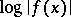is a solution of a certain non-linear elliptic partial differential equation. Forandthese equations reduce to the familiar Laplace equation and the corresponding potential theory is linear (see [a5]).

The word "quasi-regular" was introduced in this meaning in 1969 by O. Martio, S. Rickman and J. Väisälä, who found another approach to quasi-regular mappings. Their approach uses techniques and tools from the theory of spatial quasi-conformal mappings and is based, in part, on Reshetnyak's fundamental results. The tools they use in their 1969–1972 work involve the modulus of a family of curves (the extremal length) and capacities of condensers. Their work showed the power of the method of the modulus of a family of curves, made these mappings more widely known, and led to a series of important results (cf. below).

Some of the topics considered for quasi-regular mappings include:

i) stability theory, which refers to the study of-quasi-regular mappings with dilatationclose to one, see [a6];

ii) value-distribution theory, such as the analogue of the Picard theorem and its refinements, see [a7];

iii) non-linear potential theory (cf. also Potential theory) and connection of quasi-regular mappings to partial differential equations, see [a2];

iv) geometric analysis, differential forms, and applications to elasticity theory, see [a3];

v) conformal invariants and quasi-regular mappings, see [a9], [a1].

How to Cite This Entry:
Quasi-regular mapping. Encyclopedia of Mathematics. URL: http://encyclopediaofmath.org/index.php?title=Quasi-regular_mapping&oldid=34864
This article was adapted from an original article by M. Vuorinen (originator), which appeared in Encyclopedia of Mathematics - ISBN 1402006098. See original article